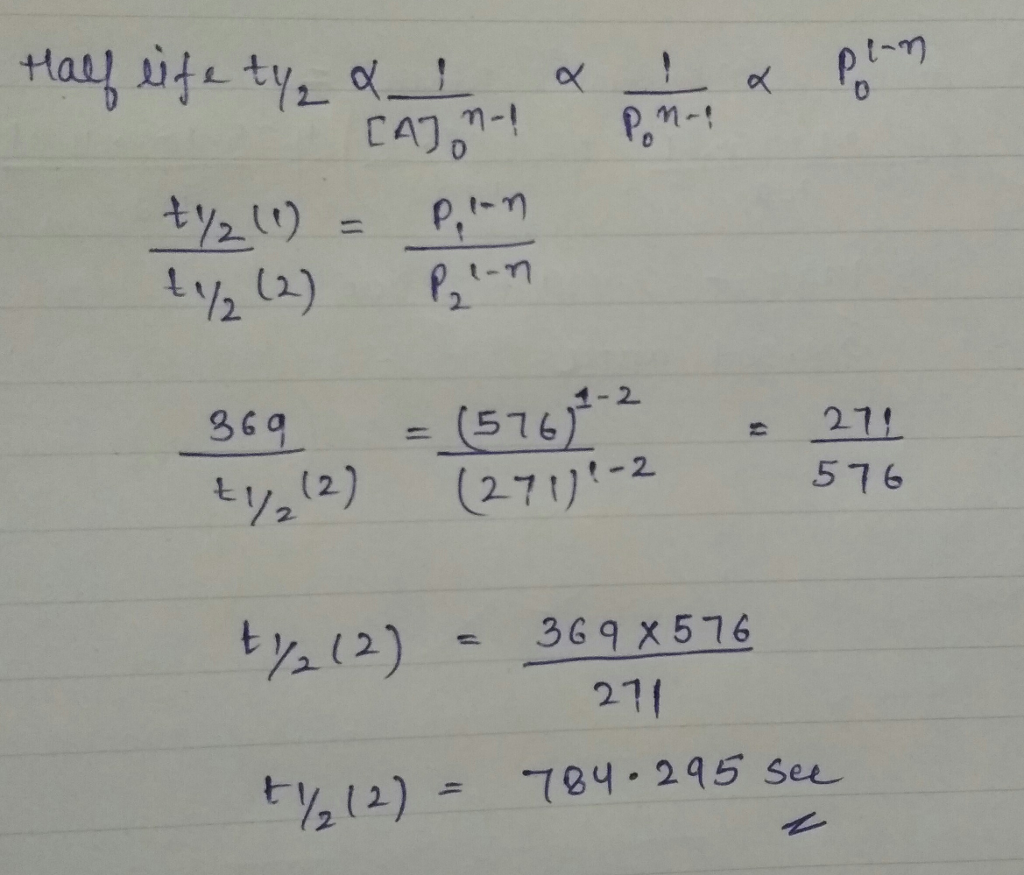# Homework Solution: The decomposition of a gaseous sample of thioketene, H2C=C=S, follows a rate law with order…

The decomposition of a gaseous sample of thioketene, H2C=C=S, follows a rate law with order 2. The half-life is 369 s when the pressure of thioketene is 576 Pa, so what is the half-life when the when the pressure is 271 Pa?